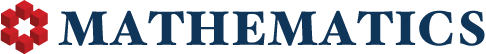## User's Total Reputation Distribution

0

(click on this box to dismiss)Q&A for people studying math at any level and professionals in related fields

``````/* http://stackoverflow.com/a/233223/3184351 */
select u.range as [prev row <= Reputation <= this row], count(*) as [Users]
from (
select case
when Reputation between 1 and 4 then 4
when Reputation between 5 and 9 then 9
when Reputation between 10 and 14 then 14
when Reputation between 15 and 19 then 19
when Reputation between 20 and 49 then 49
when Reputation between 50 and 74 then 74
when Reputation between 75 and 99 then 99
when Reputation between 100 and 124 then 124
when Reputation between 125 and 249 then 249
when Reputation between 250 and 499 then 499
when Reputation between 500 and 999 then 999
when Reputation between 1000 and 1999 then 1999
when Reputation between 2000 and 2499 then 2499
when Reputation between 2500 and 2999 then 2999
when Reputation between 3000 and 4999 then 4999
when Reputation between 5000 and 9999 then 9999
when Reputation between 10000 and 14999 then 14999
when Reputation between 15000 and 19999 then 19999
when Reputation between 20000 and 24999 then 24999
else 400000 end as range
from Users) u
group by u.range``````

### Enter Parameters

Options:
-Hold tight while we fetch your results
:records returned in :time ms:cached